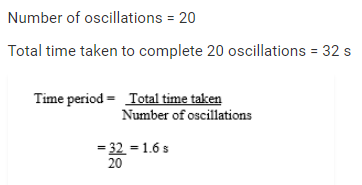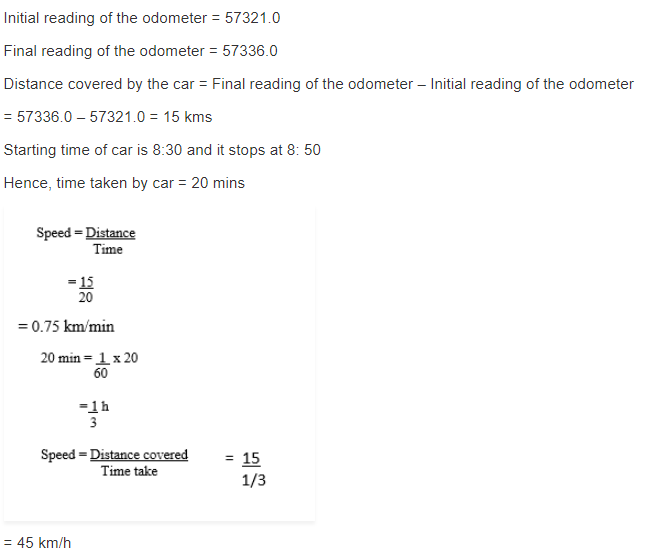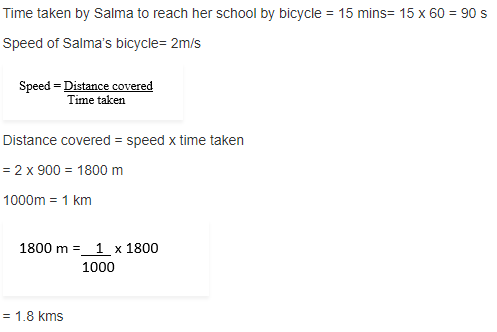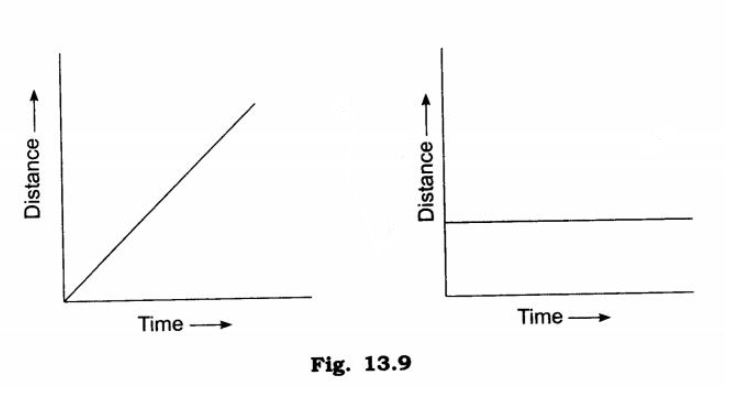# NCERT Solutions for Class 7 Science Chapter 13 Motion and Time

NCERT Solutions for Class 7 Science Chapter 13 Motion and Time

Exercises

Question:- 1. Classify the following as motion along a straight line, circular or oscillatory motion:

(i) Motion of your hands while running.

(ii) Motion of a horse pulling a cart on a straight road.

(iii) Motion of a child in a merry-go-round.

(iv) Motion of a child on a see-saw.

(v) Motion of the hammer of an electric bell.

(vi) Motion of a train on a straight bridge.

Answer:- (i) Motion of your hands while running :- Oscillatory motion

(ii) Motion of a horse pulling a cart on a straight road :- Linear motion

(iii) Motion of a child in a merry-go-round :- Circular motion

(iv) Motion of a child on a see-saw :-  Oscillatory motion

(v) Motion of the hammer of an electric bell :- Oscillatory motion

(vi) Motion of a train on a straight bridge :- Linear motion

Question:- 2. Which of the following are not correct?

(i) The basic unit of time is second.

(ii) Every object moves with a constant speed.

(iii) Distances between two cities are measured in kilometres.

(iv) The time period of a given pendulum is constant.

(v) The speed of a train is expressed in m/h.

Answer:- Incorrect statements are following:-

(ii) Every object moves with a constant speed.

(iv) The time period of a given pendulum is constant.

(v) The speed of a train is expressed in m/h.

Question:- 3. A simple pendulum takes 32 s to complete 20 oscillations. What is the time period of the pendulum?Question:- 4. The distance between two stations is 240 km. A train takes 4 hours to cover this distance. Calculate the speed of the train.

Answer:- Distance = 240 km

Time taken = 4 hours

Speed =Distance covered/time taken = 240km/4 h =60 km/h

Speed of train =60 km/h

Question:- 5. The odometer of a car reads 57321.0 km when the clock shows the time 08:30 AM. What is the distance moved by the car, if at 08:50 AM, the odometer reading has changed to 57336.0 km? Calculate the speed of the car in km/min during this time. Express the speed in km/h also.Question:- 6. Salma takes 15 minutes from her house to reach her school on a bicycle. If the bicycle has a speed of 2 m/s, calculate the distance between her house and the school.Question:- 7. Show the shape of the distance-time graph for the motion in the following cases:

(i) A car moving with a constant speed.

(ii) A car parked on a side road.Question:- 8. Which of the following relations is correct?

(i) Speed = Distance × Time            (ii) Speed = Distance/Time

(iii) Speed = Time/Distance            (iv) Speed = 1/Distance*Time

Answer:- (ii) Speed = Distance/Time

Question:- 9. The basic unit of speed is:

(i) km/min              (ii) m/min

(iii) km/h                (iv) m/s

Question:- 10. A car moves with a speed of 40 km/h for 15 minutes and then with a speed of 60 km/h for the next 15 minutes. The total distance covered by the car is:

(i) 100 km            (ii) 25 km

(iii) 15 km            (iv) 10 km

Answer:- (ii) 25 km

Question:- 11. Suppose the two photographs, shown in Fig. 13.1 and Fig. 13.2, had been taken at an interval of 10 seconds. If a distance of 100 metres is shown by 1 cm in these photographs, calculate the speed of the fastest car.

Answer:- The distance covered by the blue car (as evident from the photograph) from one horizontal white strip to another, which is measured by scale is 1.2 cm.

Given :- 1 cm = 100 m.

Therefore, 1.2 cm = 120 m.

Distance travelled by the car = 120 m

Time taken to cover this distance = Time interval between the two photographs = 10 s

Speed = distance/time

= 120/10

= 12 m/s

Question:- 12. Fig. 13.15 shows the distance-time graph for the motion of two vehicles A and B. Which one of them is moving faster?

Answer:- Vehicle A is moving faster than vehicle B.

Question:- 13. Which of the following distance-time graphs shows a truck moving with speed which is not constant?